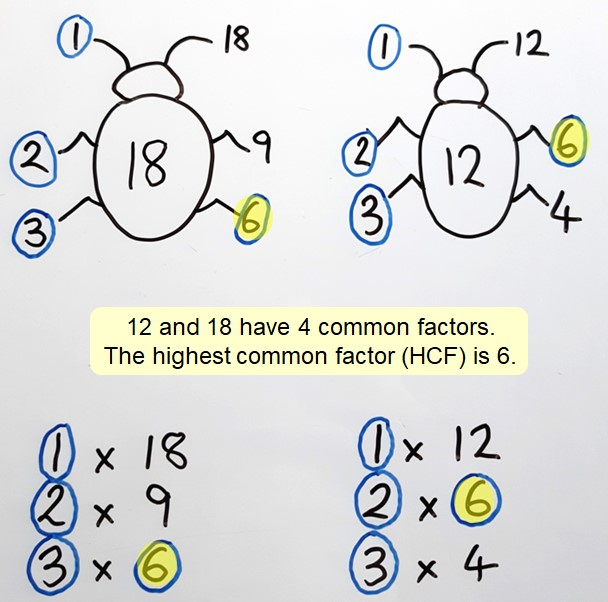## Highest common factor (HCF)

Finding the highest common factor (HCF) is useful when simplifying fractions, making the numerator and denominator smaller numbers which are easier to calculate with. This can also be referred to as finding the greatest common factor (GCF) or the greatest common divisor (GCD).

To find the highest common factor of two numbers, first record all the factor pairs for each number. Then look for the highest number that occurs in each set of pairs. This is the highest common factor.